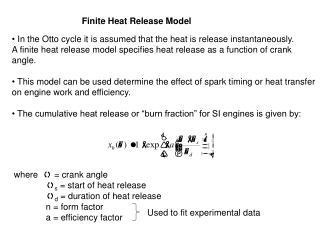DownloadDownload PresentationFinite Heat Release Model

# Finite Heat Release Model

Download Presentation## Finite Heat Release Model

- - - - - - - - - - - - - - - - - - - - - - - - - - - E N D - - - - - - - - - - - - - - - - - - - - - - - - - - -
##### Presentation Transcript

1. Finite Heat Release Model • In the Otto cycle it is assumed that the heat is release instantaneously. • A finite heat release model specifies heat release as a function of crank • angle. • This model can be used determine the effect of spark timing or heat transfer • on engine work and efficiency. • The cumulative heat release or “burn fraction” for SI engines is given by: where q = crank angle qs = start of heat release qd = duration of heat release n = form factor a = efficiency factor Used to fit experimental data

2. Finite Heat Release A typical heat release curve consists of an initial spark ignition phase, followed by a rapid burning phase and ends with burning completion phase The curve asymptotically approaches 1 so the end of combustion is defined by an arbitrary limit, such as 90% or 99% complete combustion where xb = 0.90 or 0.99 corresponding values for efficiency factor a are 2.3 and 4.6 The rate of heat release as a function of crank angle is:

3. Finite Heat Release Model

4. Finite Heat Release Model The cylinder volume in terms of crank angle, V(q), is Differentiating wrt q where For the portion of the compression and expansion strokes with no heat Release, where q < qs and q > qs + qd dQ/dq = 0 and

5. Finite Heat Release Model Results Start of heat release: Engine 1 - 20o bTC Engine 2 - TC Duration 40o

6. Finite Heat Release Model Results